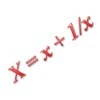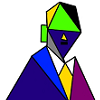# Resources tagged with: Transformation of functions

Filter by: Content type:
Age range:
Challenge level:

### There are 12 results

Broad Topics > Coordinates, Functions and Graphs > Transformation of functions### Cubic Spin

##### Age 16 to 18Challenge Level

Prove that the graph of f(x) = x^3 - 6x^2 +9x +1 has rotational symmetry. Do graphs of all cubics have rotational symmetry?### The Why and How of Substitution

##### Age 16 to 18

Step back and reflect! This article reviews techniques such as substitution and change of coordinates which enable us to exploit underlying structures to crack problems.### Agile Algebra

##### Age 16 to 18Challenge Level

Observe symmetries and engage the power of substitution to solve complicated equations.### Parabolas Again

##### Age 14 to 18Challenge Level

Here is a pattern composed of the graphs of 14 parabolas. Can you find their equations?### Exploring Cubic Functions

##### Age 14 to 18Challenge Level

Quadratic graphs are very familiar, but what patterns can you explore with cubics?### Sine Problem

##### Age 16 to 18Challenge Level

In this 'mesh' of sine graphs, one of the graphs is the graph of the sine function. Find the equations of the other graphs to reproduce the pattern.### Parabolic Patterns

##### Age 14 to 18Challenge Level

The illustration shows the graphs of fifteen functions. Two of them have equations y=x^2 and y=-(x-4)^2. Find the equations of all the other graphs.### More Parabolic Patterns

##### Age 14 to 18Challenge Level

The illustration shows the graphs of twelve functions. Three of them have equations y=x^2, x=y^2 and x=-y^2+2. Find the equations of all the other graphs.### Ellipses

##### Age 14 to 18Challenge Level

Here is a pattern for you to experiment with using graph drawing software. Find the equations of the graphs in the pattern.### Operating Machines

##### Age 16 to 18Challenge Level

What functions can you make using the function machines RECIPROCAL and PRODUCT and the operator machines DIFF and INT?### Painting by Functions

##### Age 16 to 18Challenge Level

Use functions to create minimalist versions of works of art.### Loch Ness

##### Age 16 to 18Challenge Level

Draw graphs of the sine and modulus functions and explain the humps.